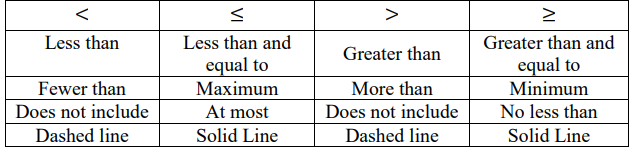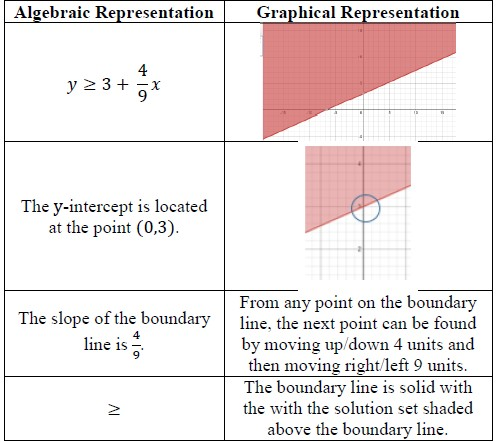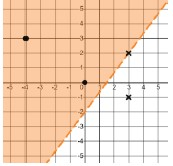# MA.912.AR.2.8Export Print
Given a mathematical or real-world context, graph the solution set to a two-variable linear inequality.

### Clarifications

Clarification 1: Instruction includes the use of standard form, slope-intercept form and point-slope form and any inequality symbol can be represented.

Clarification 2: Instruction includes cases where one variable has a coefficient of zero.

General Information
Subject Area: Mathematics (B.E.S.T.)
Strand: Algebraic Reasoning
Status: State Board Approved

## Benchmark Instructional Guide

### Terms from the K-12 Glossary

• Coordinate Plane
• Linear Expression

### Vertical Alignment

Previous Benchmarks

Next Benchmarks

### Purpose and Instructional Strategies

In grade 8, students graphed linear two-variable equations. In Algebra I, students graph the solution set to a two-variable linear inequality. In later courses, students will solve problems involving linear programming and will graph the solutions sets of two-variable quadratic inequalities.
• Instruction includes the use of linear inequalities in standard form, slope-intercept form and point-slope form. Include examples in which one variable has a coefficient of zero such as $x$ < −$\frac{\text{17}}{\text{5}}$.
• Instruction includes the connection to graphing solution sets of one-variable inequalities on a number line; recognizing whether the boundary line should be dotted (exclusive) or solid (inclusive). Additionally, have students use a test point to confirm which side of the line should be shaded (MTR.6.1).
• Students should recognize that the inequality symbol only directs where the line is shaded (above or below) for inequalities when in slope-intercept form. Students shading inequalities in other forms will need to use a test point to determine the correct half-plane to shade.

### Common Misconceptions or Errors

• Students often choose to shade to wrong half-plane when graphing two-variable linear inequalities.
• Students may think that the inequality symbol’s orientation always determines the side of the line to shade.
• For example, students may say that inequalities with a less than symbol should be shaded below the line while inequalities with a greater than symbol should be shaded above the line. This typically happens after graphing multiple inequalities in slope-intercept form. To address this, provides counterexamples to this such as 3$x$ − 2$y$ < 15 $o$$r$ − 4$x$ − 7 ≥ $y$. Use these counterexamples to emphasize the benefit of using a test point to confirm the direction of shading.

### Strategies to Support Tiered Instruction

• Instruction includes opportunities to use a highlighter to identify the phrases “is less than,” “is greater than,” “is less than or equal to,” and “is greater than or equal to” when writing inequalities.
• Teacher provides instruction modeling how to correctly identify the solution set of a linear inequality given in slope-intercept form. After graphing, students can circle the y intercept. If the inequality is in form $y$ < $m$$x$ + $b$ or $y$$m$$x$ + $b$, the solution set is the half-plane that contains the $y$-axis values below the $y$-intercept. If the inequality is in form $y$ > $m$$x$ + $b$ or $y$$m$$x$ + $b$, the solution set is the half-plane that contains the $y$- axis values above the $y$-intercept.
• Instruction includes opportunities to graph the boundary line of a system of inequalities, based on an inaccurate translation from word problem. To assist in determining the boundary line for the system, students can create a graphic organizer like the one below.• Instruction includes making the connection between the algebraic and graphical representations of a two-variable linear inequality and its key features.
• For example, teacher can provide a graphic organizer such as the one below.• Instruction includes opportunities to identify a test point to plug into an inequality. It is usually easiest to use the origin (0,0) as it makes mental calculations easier. If the point selected creates a true statement, their inequality is true and they should shade in the half-plane containing that point. If it creates a false statement, they should shade in the half-plane not containing that point. By using a test point, students avoid the mistake of thinking that the direction of the inequality determines the shading.
• For example, the points (−4,3), (0,0), (3,2) and (3, −1) were used to determine where to shade for the inequality 4$x$ − 3$y$ < 6 shown below.• Penelope is planning to bake cakes and cookies to sell for an upcoming school fundraiser.
• Each cake requires 1$\frac{\text{3}}{\text{4}}$ cups of flour and each batch of cookies requires 2$\frac{\text{1}}{\text{4}}$ cups of flour.
• Penelope bought 3 bags of flour. Each bag contains around 17 cups of flour.
• Part A. Assuming she has all the other ingredients needed, create a graph to show all the possible combinations of cakes and batches of cookies Penelope could make.
• Part B. Create constraints for this given situation.

### Instructional Items

Instructional Item 1
• Graph the solution set to the inequality $y$ + 3 > −2($x$ − 2).

*The strategies, tasks and items included in the B1G-M are examples and should not be considered comprehensive.

## Related Courses

This benchmark is part of these courses.
1200310: Algebra 1 (Specifically in versions: 2014 - 2015, 2015 - 2022, 2022 and beyond (current))
1200320: Algebra 1 Honors (Specifically in versions: 2014 - 2015, 2015 - 2022, 2022 and beyond (current))
1200370: Algebra 1-A (Specifically in versions: 2014 - 2015, 2015 - 2022, 2022 and beyond (current))
1200400: Foundational Skills in Mathematics 9-12 (Specifically in versions: 2014 - 2015, 2015 - 2022, 2022 and beyond (current))
7912080: Access Algebra 1A (Specifically in versions: 2014 - 2015, 2015 - 2018, 2018 - 2019, 2019 - 2022, 2022 and beyond (current))
1200315: Algebra 1 for Credit Recovery (Specifically in versions: 2014 - 2015, 2015 - 2022, 2022 and beyond (current))
1200375: Algebra 1-A for Credit Recovery (Specifically in versions: 2014 - 2015, 2015 - 2022, 2022 and beyond (current))
7912075: Access Algebra 1 (Specifically in versions: 2014 - 2015, 2015 - 2018, 2018 - 2019, 2019 - 2022, 2022 and beyond (current))
1209315: Mathematics for ACT and SAT (Specifically in versions: 2022 and beyond (current))

## Related Access Points

Alternate version of this benchmark for students with significant cognitive disabilities.
MA.912.AR.2.AP.8: Given a two-variable linear inequality, select a graph that represents the solution.

## Related Resources

Vetted resources educators can use to teach the concepts and skills in this benchmark.

## Formative Assessments

Linear Inequalities in the Half-Plane:

Students are asked to graph all solutions for a non-strict <= or >= linear inequality in the coordinate plane.

Type: Formative Assessment

Graphing Linear Inequalities:

Students are asked to graph a strict < or > linear inequality in the coordinate plane.

Type: Formative Assessment

## Original Student Tutorial

Graphing Linear Inequalities:

Learn to graph linear inequalities in two variables to display their solutions as you complete this interactive tutorial.

Type: Original Student Tutorial

## MFAS Formative Assessments

Graphing Linear Inequalities:

Students are asked to graph a strict < or > linear inequality in the coordinate plane.

Linear Inequalities in the Half-Plane:

Students are asked to graph all solutions for a non-strict <= or >= linear inequality in the coordinate plane.

## Original Student Tutorials Mathematics - Grades 9-12

Graphing Linear Inequalities:

Learn to graph linear inequalities in two variables to display their solutions as you complete this interactive tutorial.

## Student Resources

Vetted resources students can use to learn the concepts and skills in this benchmark.

## Original Student Tutorial

Graphing Linear Inequalities:

Learn to graph linear inequalities in two variables to display their solutions as you complete this interactive tutorial.

Type: Original Student Tutorial

## Parent Resources

Vetted resources caregivers can use to help students learn the concepts and skills in this benchmark.